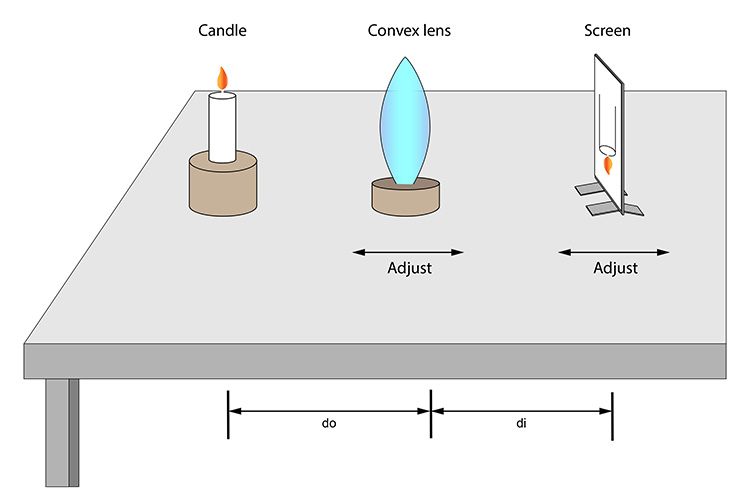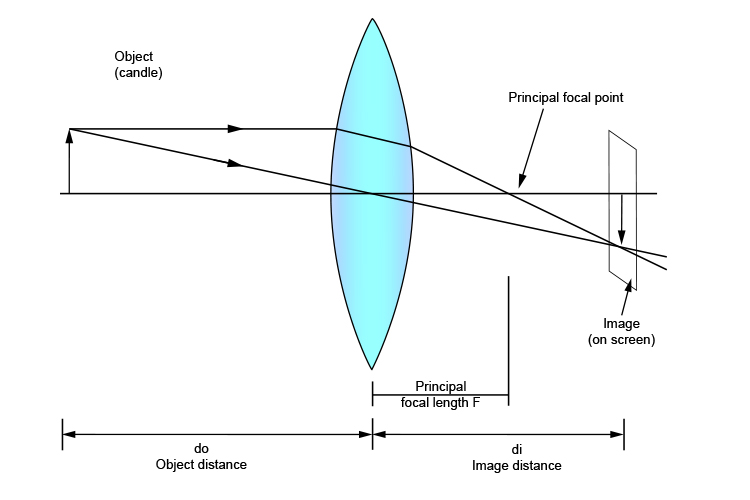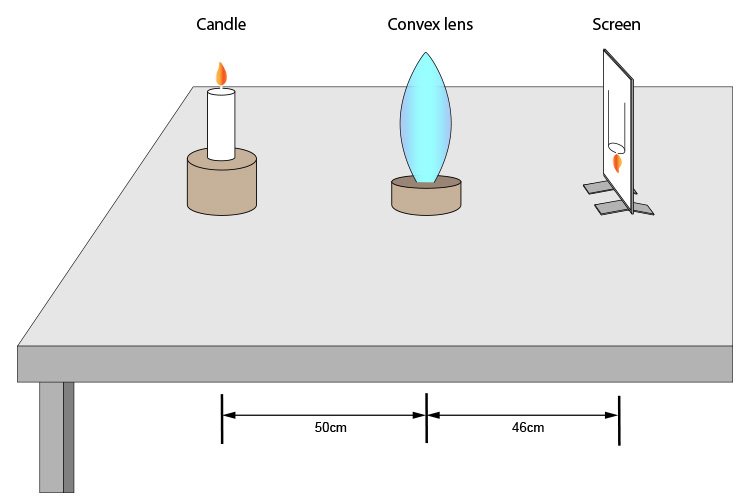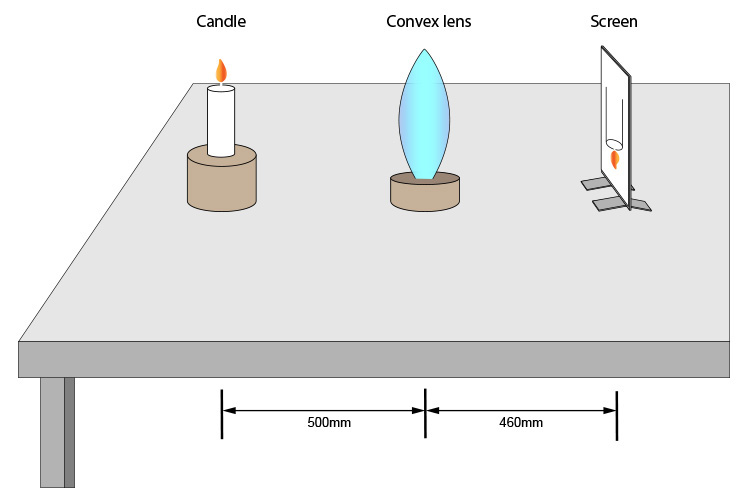# Practical ways to find the principal focal length - lens equation

There is another way to find the principal focal length of a convex lens but unfortunately, we have to learn another equation, the lens equation.

But it is really easy to remember using the following mnemonic:

Lens equation
IF I DO I DIE

I/F=I/(do)+I/(di)

1/F=1/(do)+1/(di)

The lens equation is:

1/F=1/(do)+1/(di)

Where

 F = Principal focal length do = Object distance di = Image distance

Place a burning candle at one end of a table, a convex lens in the centre of the table and a screen at the other end of the table.

Move the screen and convex lens backwards and forwards on the table until a sharp upside-down image of the burning candle is made.Once you have a sharp upside-down image on the screen you can measure do and di and put the figures into the formula.

1/F=1/(do)+1/(di)

and work out the principal focal point F.

In ray diagram format that would be:An example would be:

Question 1

In the experiment below an upside down image of the candle has been clearly formed on the screen. What is the convex lens's principal focal length?Using the lens equation we have

1/F=1/(do)+1/(di)

1/F=1/50+1/46

1/F=0.02+0.21739

1/F=0.041739

F=1/0.041739

F=23.96cm

Question 2

Just to show you that this formula works in any unit, let's do the same experiment but measure the distances in mm. What is the principal focal length?Using the lens equation we have.

1/F=1/(do)+1/(di)

1/F=1/500+1/460

1/F=0.0041739

F=1/0.0041739

F=239.58mm